Question

# 2Al + 3CuCl2 —> 2AlCl3 + 3Cu Oxidation Numbers Reproduce the following table in your document....

2Al + 3CuCl2 —> 2AlCl3 + 3Cu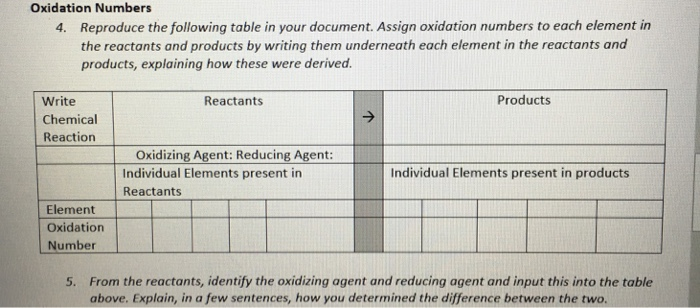Oxidation Numbers Reproduce the following table in your document. Assign oxidation numbers to each element in the reactants and products by writing them underneath each element in the reactants and products, explaining how these were derived. 4. Products Write Chemical Reaction Reactants Oxidizing Agent: Reducing Agent Individual Elements present in products Individual Elements present in Reactants Element Oxidation Number From the reactants, identify the oxidizing agent and reducing agent and input this into the table above. Explain, in a few sentences, how you determined the difference between the two. 5.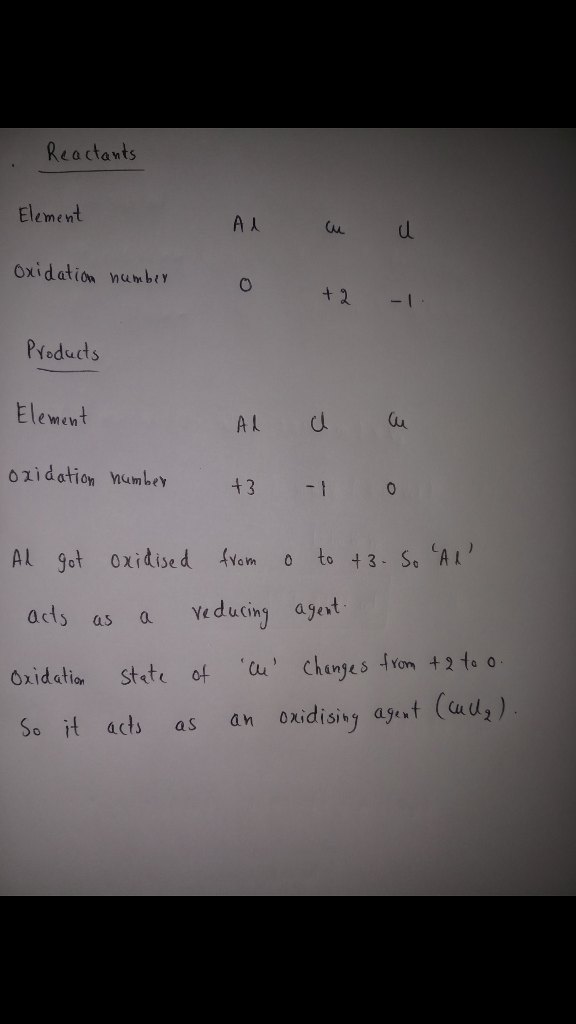#### Earn Coins

Coins can be redeemed for fabulous gifts.

Similar Homework Help Questions
• ### The reducing agent in the reaction below is 3CuCl2(aq)+2Al(s) →3Cu(s)+2AlCl3(aq) a. Al3+ b. Cu2+ c. Al...

The reducing agent in the reaction below is 3CuCl2(aq)+2Al(s) →3Cu(s)+2AlCl3(aq) a. Al3+ b. Cu2+ c. Al d. Cu

• ### Chem 2A: Oxidation Numbers, Oxidation/Reduction rium 1. Determine the oxidation number of chlorine in the f...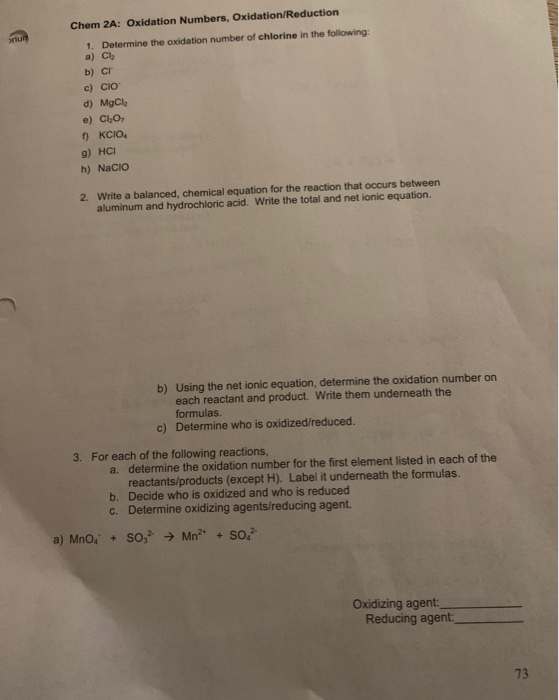Chem 2A: Oxidation Numbers, Oxidation/Reduction rium 1. Determine the oxidation number of chlorine in the following: a) Cl b) C c) CIO d) MgCl e) ClO ) KCIO g) HCI h) NaCIO 2. Write a balanced, chemical equation for the reaction that occurs between aluminum and hydrochloric acid. Write the total and net ionic equation. b) Using the net ionic equation, determine the oxidation number on each reactant and product. Write them underneath the formulas. Determine who is oxidized/reduced. c)...

• ### Assign oxidation numbers to all of the atoms of each element in the following reaction a) Cu(s) + NO3-1(aq) => &...

Assign oxidation numbers to all of the atoms of each element in the following reaction a) Cu(s) + NO3-1(aq) =>   Cu+2(aq) + NO(g) The oxidizing agent in this reaction is_________________ The reducing agent is this reaction is_________________

• ### Name Date Class Redox Worksheet #1 - Assigning Oxidation Numbers Instructions: A. Assign oxidation numbers to...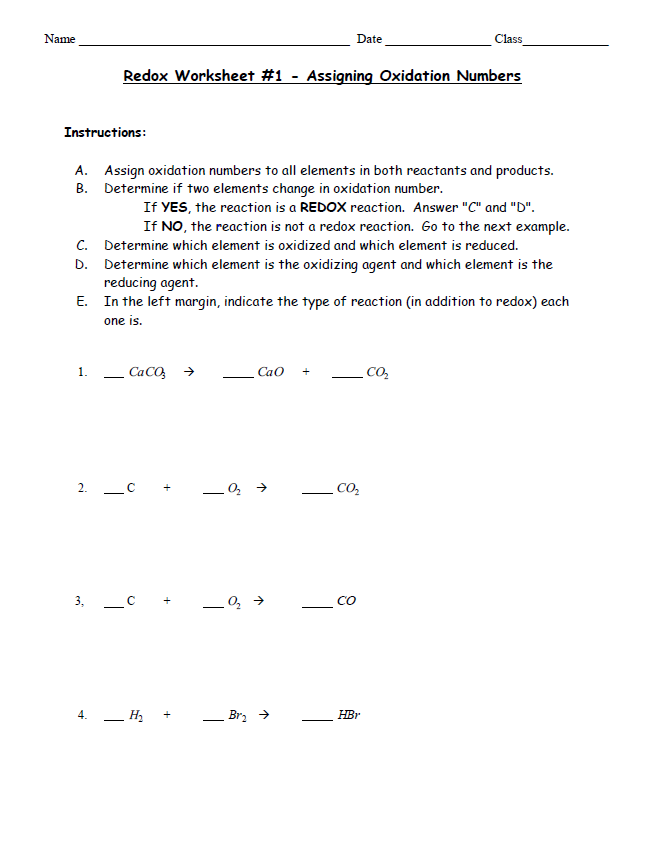Name Date Class Redox Worksheet #1 - Assigning Oxidation Numbers Instructions: A. Assign oxidation numbers to all elements in both reactants and products. B. Determine if two elements change in oxidation number. If YES, the reaction is a REDOX reaction. Answer "C" and "D". If NO, the reaction is not a redox reaction. Go to the next example. C. Determine which element is oxidized and which element is reduced. D. Determine which element is the oxidizing agent and which element...

• ### Instructions: Assign oxidation numbers for each individual atom in all of the substances below by writing...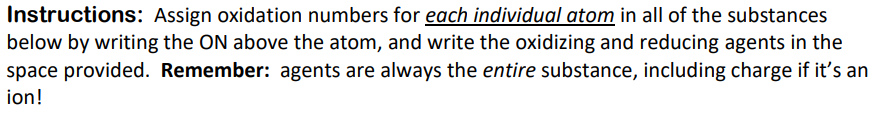Instructions: Assign oxidation numbers for each individual atom in all of the substances below by writing the ON above the atom, and write the oxidizing and reducing agents in the space provided. Remember: agents are always the entire substance, including charge if it's an ion! Fe3+(aq) + Sn2+(aq) → Fe2+(aq) + Sn4+(aq) Ox agent: Red agent: HNO2(aq) + MnO4 (aq) + Mn2+(aq) + NO3(aq) Ox agent: Red agent: SO32 (aq) + MnO4 (aq) → SO42"aq) + MnO2(aq) (not peroxide) Ox...

• ### Іго, Electrochemistry Wolleet 1. Assign oxidation numbers to each element in the following compounds: a. VOCI...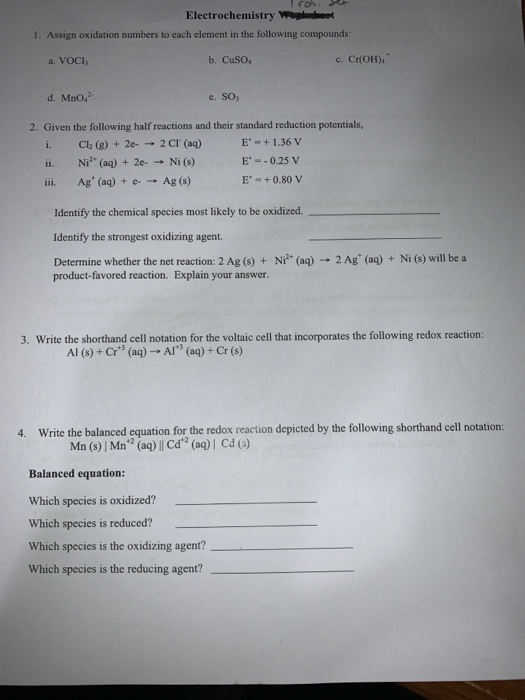Іго, Electrochemistry Wolleet 1. Assign oxidation numbers to each element in the following compounds: a. VOCI b. Cuso c. CHOH). d. Mno, e. SO, 2. Given the following half reactions and their standard reduction potentials, i. Cl (g) + 2e- - 2 CI (aq) E' =+ 1.36 V ii. Niº (aq) + 2e - Ni (s) E' = -0.25 V iii. Ag (aq) + e- Ag () E' = +0.80 V Identify the chemical species most likely to be oxidized....